# Pre-Algebra : Multiplicative Inverse Property

## Example Questions

### Example Question #1 : Multiplicative Inverse Property

Simplify.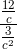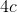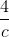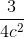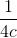Explanation:

To simplify a compound fraction, multiply the numerator by the reciprocal of the denominator. Remember that a compound fraction can easily be rewritten as a division problem!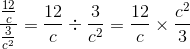Solve the multiplication.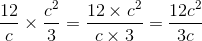Now we need to reduce the fraction to find our final answer.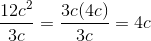### Example Question #1 : Multiplicative Inverse Property

What is the multiplicative inverse of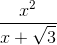where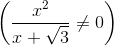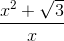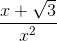Explanation:

The rule for Multiplicative Inverse Property is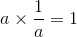where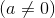.

Using this rule, if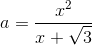,

then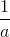is the Mulitplicative inverse, which is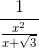.

After you simplify you getwhich is the Multiplicative Inverse.

### Example Question #1 : Multiplicative Inverse Property

What is the multiplicative inverse of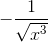where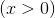?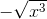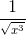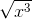Explanation:

The rule for Multiplicative Inverse Property iswhere.

Using this rule, if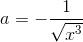,

thenis the  mulitplicative inverse, which is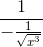.

After you simplify you getwhich is the multiplicative inverse.

### Example Question #1 : Multiplicative Inverse Property

Which of the following statements demonstrates the inverse property of multiplication?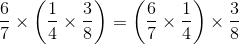None of the examples in the other responses demonstrates the inverse property of multiplication.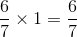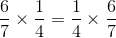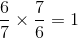Explanation:

The inverse property of multiplication states that for every real number, a number exists, called the multiplicative inverse, such that the number and its inverse have product 1. Of the statements given, onlydemonstrates this property.

### Example Question #1 : Multiplicative Inverse Property

Which of the following displays the multiplicative inverse property?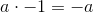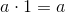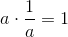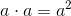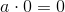Explanation:

The mulitplicative inverse property deals with reciprocals.  For example, the multiplicative inverse, or reciprocal, of the number 7 is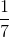.

The multiplicative inverse property states that a number times its multiplicative inverse equals 1.

Therefore,displays the multiplicative inverse property.

### All Pre-Algebra Resources